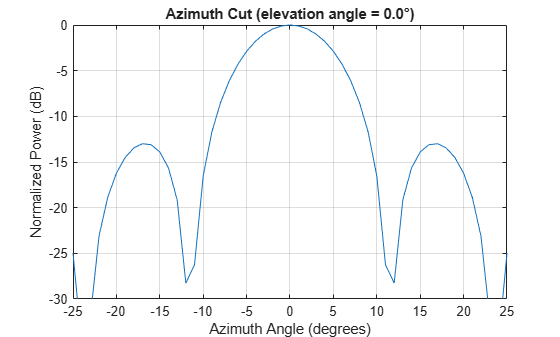# Super-Resolution DOA Estimation

This example shows how to estimate angles of arrival from two separate signal sources when both angles fall within the main lobe of the array response a uniform linear array (ULA). In this case, a beamscan DOA estimator cannot resolve the two sources. However, a super-resolution DOA estimator using the root MUSIC algorithm is able to do so.

Plot the array response of the ULA. Zoom in on the main lobe.

```fc = 1e9; lambda = physconst('LightSpeed')/fc; array = phased.ULA('NumElements',10,'ElementSpacing',lambda/2); array.Element.FrequencyRange = [8e8 1.2e9]; plotResponse(array,fc,physconst('LightSpeed')) axis([-25 25 -30 0]);```Receive two signal sources with DOAs separated by 10°.

```ang1 = [30; 0]; ang2 = [40; 0]; Nsnapshots = 1000; rng default npower = 0.01; rxsig = sensorsig(getElementPosition(array)/lambda,... Nsnapshots,[ang1 ang2],npower);```

Estimate the directions of arrival using the beamscan estimator. Because both DOAs fall inside the main lobe of the array response, the beamscan DOA estimator cannot resolve them as separate sources.

```beamscanestimator = phased.BeamscanEstimator('SensorArray',array,... 'OperatingFrequency',fc,'ScanAngles',-90:90,... 'DOAOutputPort',true,'NumSignals',2); [~,sigang] = beamscanestimator(rxsig); plotSpectrum(beamscanestimator)```Use the super-resolution DOA estimator to estimate the two directions. This estimator offers better resolution than the nonparametric beamscan estimator.

```MUSICestimator = phased.RootMUSICEstimator('SensorArray',array,... 'OperatingFrequency',fc,'NumSignalsSource','Property',... 'NumSignals',2,'ForwardBackwardAveraging',true); doa_est = MUSICestimator(rxsig)```
```doa_est = 1×2 40.0091 30.0048 ```

This estimator correctly identifies the two distinct directions of arrival.